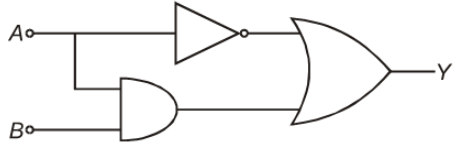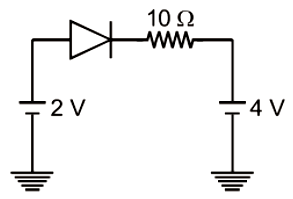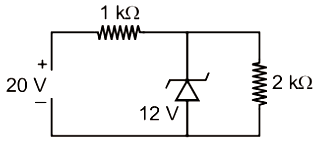The output Y of the logic gate circuit shown in figure is best represented as(1) $\overline{)A}+\left(A.B\right)$

(2) $A+\overline{)\left(A+B\right)}$

(3) A+(A.B)

(4) $\overline{)A}+\overline{)\left(A.B\right)}$

Concept Questions :-

Logic gates
High Yielding Test Series + Question Bank - NEET 2020

Difficulty Level:

A transistor is used in common emitter mode in an amplifier circuit. When a signal of 10 mV is added to the base-emitter voltage, the base current changes by 15 $\mu$A and the collector current changes by 1.5 mA. The current gain b will be

1.  50

2.  48

3.  100

4.  200

Concept Questions :-

Applications of Transistor
High Yielding Test Series + Question Bank - NEET 2020

Difficulty Level:

The current through an ideal p-n junction diode shown in the circuit will be -1.  5 A

2.  0.2 A

3.  0.6 A

4.  Zero

Concept Questions :-

Applications of PN junction
High Yielding Test Series + Question Bank - NEET 2020

Difficulty Level:

In a common-emitter transistor, the phase difference between input and output is:

1.  Zero

2.  $\mathrm{\pi }$/2

3.  $\mathrm{\pi }$/3

4.  $\mathrm{\pi }$

Concept Questions :-

Transistor
High Yielding Test Series + Question Bank - NEET 2020

Difficulty Level:1.  36 mW

2.  12 mW

3.  144 mW

4.  64 mW

Concept Questions :-

Applications of PN junction
High Yielding Test Series + Question Bank - NEET 2020

Difficulty Level:

1.  N-type

2.  P-type

3.  intrinsic

4.  insulator

Concept Questions :-

Types of semiconductors
High Yielding Test Series + Question Bank - NEET 2020

Difficulty Level:

When the temperature of the silicon sample is increased from  to , the conductivity of silicon will be

1.  Increased

2.  Decreased

3.  Remain same

4.  Zero

Concept Questions :-

Types of semiconductors
High Yielding Test Series + Question Bank - NEET 2020

Difficulty Level:

The conductivity of an n-type semiconductor whose density of conduction electrons is ${\mathrm{n}}_{\mathrm{e}}$, Density of holes is ${\mathrm{n}}_{\mathrm{h}}$, Mobility of conduction electrons is ${\mathrm{m}}_{\mathrm{e}}$and Mobility of holes is ${\mathrm{m}}_{\mathrm{h}},$ will be

1.

2.

3.

4.

Concept Questions :-

Types of semiconductors
High Yielding Test Series + Question Bank - NEET 2020

Difficulty Level:

In semiconductors, which of the following gives the law of mass action (where symbols have their usual meanings)-

1.

2.

3.

4.

Concept Questions :-

Types of semiconductors
High Yielding Test Series + Question Bank - NEET 2020

Difficulty Level:

For a transistor amplifier, the power gain and voltage gain are 150 and 10 respectively. The current gain is-

1.

2.  $\frac{1}{15}$

3.  15

4.  1500

Concept Questions :-

Applications of Transistor